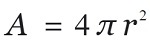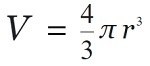# Rust program to calculate the Surface Area and Volume of Sphere

Given the radius of the Sphere, we have to calculate the Surface Area and Volume of the Sphere using the Rust program.
Submitted by Nidhi, on October 01, 2021

Problem Solution:

Here, we will read the radius from the user. Then we will calculate the surface area and volume of Sphere and print the result.

The surface area of the Sphere:

The formula to calculate the surface area of the Sphere is:The volume of the Sphere:

The formula to calculate the volume of the Sphere is:Program/Source Code:

The source code to calculate the surface area, volume of the Cone is given below. The given program is compiled and executed successfully.

```// Rust program to calculate the
// Surface Area and Volume of Sphere

use std::io;

fn main()
{

let mut area:f32    =0.0;
let mut volume:f32  =0.0;

let mut input = String::new();

radius = input.trim().parse().expect("Not a valid number");

println!("Volume of Sphere 	    : {}", volume);
println!("Surface area of Sphere: {}", area);
}
```

Output:

```RUN 1:
3.5
Volume of Sphere            : 179.50334
Surface area of Sphere: 153.86002

RUN 2:
12.3
Volume of Sphere            : 7790.831
Surface area of Sphere: 1900.2025
```

Explanation:

Here, we read the radius from the user. Then we calculated the surface area, volume of the Sphere and printed the result.